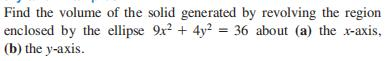### Still have math questions?

Trigonometry
QuestionFind the volume of the solid generated by revolving the region enclosed by the ellipse $$9 x ^ { 2 } + 4 y ^ { 2 } = 36$$ about (a) the $$x$$ -axis,

(b) the $$y$$ -axis.

$$\left. \begin{array} { l l } { \text { (a) } 24 \pi } & { \text { (b) } 16 \pi } \end{array} \right.$$